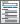Home  /  Products  /  Features  /  Basic statistics

## Basic statistics

Summaries

Tables and tabulations• Over groups and/or variables
• Statistics may include mean, count, sum, min, max, range, standard deviation, variance, variation coefficient, standard error of mean, skewness, kurtosis, median, percentiles, and interquartile range
• Results from any summary-statistics commandCreation of datasets of summary statistics• Statistics by group or subgroup of observations
• Statistics: mean, standard deviation, count, min, max, median, percentiles, and interquartile range
• Pairwise differences in means with tests and confidence intervals
• Multiple-comparison adjustments: Bonferroni, Šidák, Scheffé, Tukey HSD, Duncan, and Student–Newman–Keuls adjustments• Group comparisons that are significant
• Balanced and unbalanced data
• One sample
• Two independent samples
• Two paired samples
• Based on summary data (ttesti)

Tests of proportions• Comparison of means
• Cohen's d
• Hedge's g
• Glass's Δ
• Point/biserial correlation
• Confidence intervals
• Variance explained by regression and ANOVA
• Eta-squared—η2
• Omega-squared—ω2
• Confidence intervals

Other common tests

Correlations

• Automatically create indicators based on categorical variables
• Form interactions among discrete and continuous variables
• Include polynomial terms
• Perform contrasts of categories/levels
Watch Introduction to Factor Variables in Stata tutorials